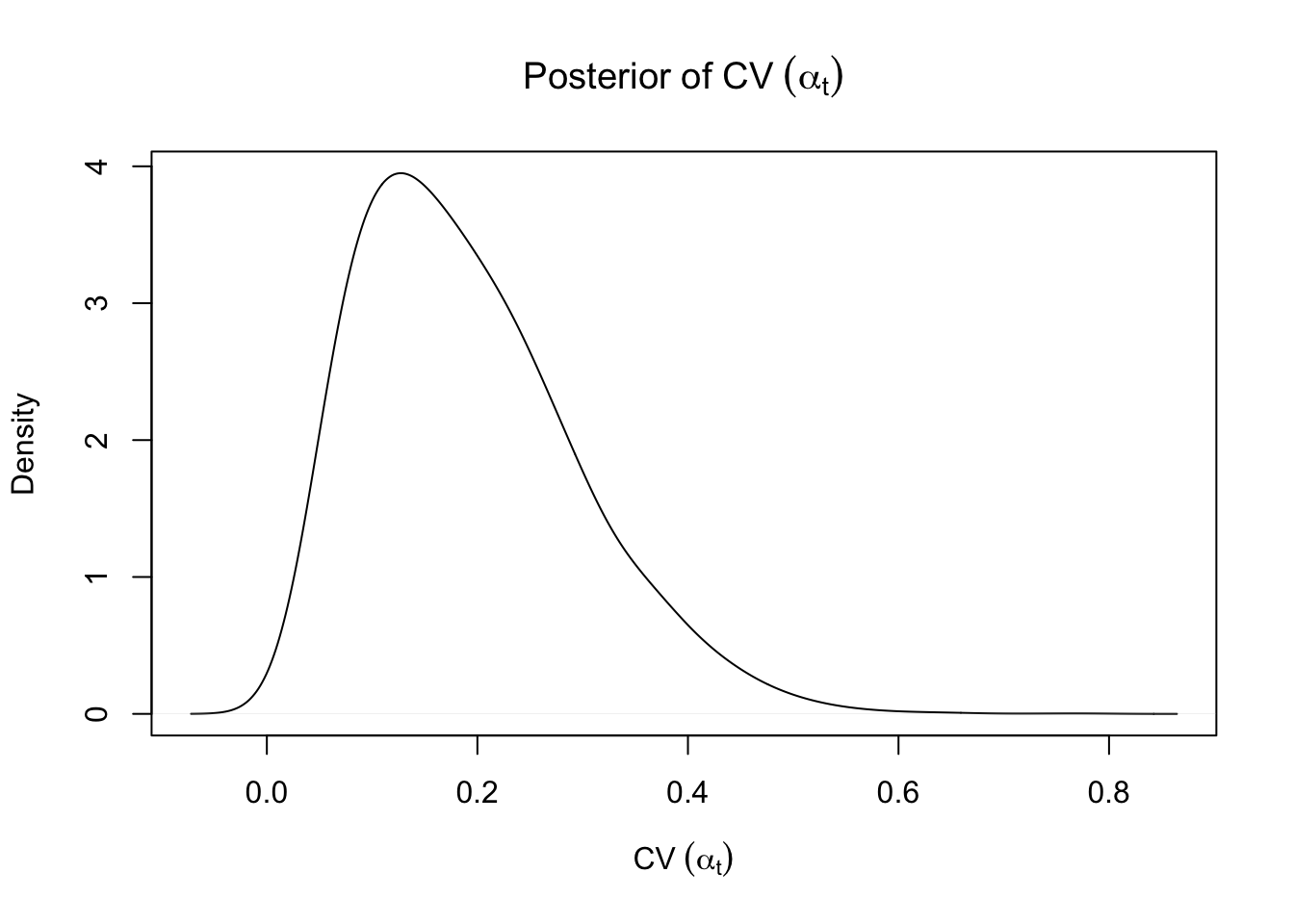# Statistics in Glaucoma: Part II

by Sam Berchuck and Joshua Warren

Samuel Berchuck is a Postdoctoral Associate in Duke University’s Department of Statistical Science and Forge-Duke’s Center for Actionable Health Data Science.

Joshua L. Warren is an Assistant Professor of Biostatistics at Yale University.

## Analyzing Visual Field Data

In Part I of this series on statistic in glaucoma, we detailed the use of visual fields for understanding functional vision loss in glaucoma patients. Before discussing a new method for modeling visual field data that accounts for the anatomy of the eye, we discussed how visual field data is typically analyzed by introducing a common diagnostic metric, point-wise linear regression (PLR). PLR is a trend-based diagnostic that uses slope p-values from the location specific linear regressions to discriminate progression status. The motivation for PLR is straightforward, assuming that large negative slopes at numerous visual field locations is indicative of progression. This is characteristic of a large class of methods for analyzing visual field data that attempt to discriminate progression based on changes in the DLS across time. This technique is simple, intuitive, and effective; however, it is often limited due to the naivete of modeling assumptions, including the independence of visual field locations.

## Ocular Anatomy in the Neighborhood Structure of the Visual Field

To properly account for the spatial dependencies on the visual field, Berchuck et al. 2018 introduce a neighborhood model that incorporates anatomical information through a dissimilarity metric. Details of the method can be found in Berchuck et al. 2018, but we provide a quick introduction. The key development is the specification of the neighborhood structure through a new definition of adjacency weights. Typically in areal data, the adjacency for two locations $$i$$ and $$j$$ is defined as $$w_{ij} = 1(i \sim j)$$, where $$i \sim j$$ is the event that locations $$i$$ and $$j$$ are neighbors. As discussed in Part I, this assumption is not sufficient due to the complex anatomy of the eye. To account for this additional structure, a more general adjacency is introduced that is a function of a dissimilarity metric, $$w_{ij}(\alpha_t) = 1(i \sim j)\exp\{-z_{ij}\alpha_t\}$$. Here, $$z_{ij}$$ is a dissimilarity metric that represents the absolute difference between the Garway-Heath angles of locations $$i$$ and $$j$$.

The parameter $$\alpha_t$$ dictates the importance of the dissimilarity metric at each visual field exam $$t$$. When $$\alpha_t$$ becomes large, the model reduces to an independent process, and as $$\alpha_t$$ goes to zero, the process becomes the standard spatial model for areal data. Based on the specification of the adjacency weights, $$\alpha_t$$ has a useful interpretation with respect to deterioration of visual ability. In particular, $$\alpha_t$$ changing over exams indicates that the neighborhood structure on the visual field is changing, which in turn implies damage to the underlying retinal ganglion cell structure. This observation motivates a diagnostic of progression that quantifies variability in $$\alpha_t$$ across time. We choose the coefficient of variation (CV) and demonstrate that is a highly significant predictor of progression, and furthermore, independent of trend-based methods such as PLR.

## Converting MCMC Samples into Clinical Statements

Now we calculate the posterior distribution of the CV of $$\alpha_t$$ and print its moments.

CVAlpha <- apply(Alpha, 1, function(x) sd(x) / mean(x))
plot(density(CVAlpha, adjust = 2), main = expression("Posterior of CV"~(alpha[t])), xlab = expression("CV"~(alpha[t])))STCV <- c(mean(CVAlpha), sd(CVAlpha), quantile(CVAlpha, probs = c(0.025, 0.975)))
names(STCV)[1:2] <- c("Mean", "SD")
print(STCV)
##       Mean         SD       2.5%      97.5%
## 0.19121622 0.10205826 0.04636219 0.42744656

For this information to be useful clinically, we convert it into a probability of progression based on a model trained on a large cohort of glaucoma patients (Berchuck et al. 2019). Because the information from $$\alpha_t$$ is independent of trend-based methods, we show that the optimal use of $$\alpha_t$$ is combining it with a basic global metric that includes the slope and p-value (and their interaction) of the overall mean at each visual field exam. The trained model coefficients are publicly available and are used below. Furthermore, both the mean, standard deviation, and their interaction of the CV of $$\alpha_t$$ are included. The probability of progression can be calculated as follows.

###Calculate the global metric slope and p-value
MeanSens <- apply(t(matrix(VFSeries$DLS, ncol = Nu)) / 10, 1, mean) # scaled mean DLS reg.global <- lm(MeanSens ~ Time) # global regression GlobalS <- summary(reg.global)$coef[2, 1] # global slope
GlobalP <- summary(reg.global)\$coef[2, 4] # global p-value

###Obtain probabiltiy of progression using estimated parameters from Berchuck et al. 2019
input <- c(1, GlobalP, GlobalS, STCV, STCV, GlobalS * GlobalP, STCV * STCV)
coef <- c(-1.7471655, -0.2502131, -13.7317622, 7.4746348, -8.9152523, 18.6964153, -13.3706058)
fit <- input %*% coef
exp(fit) / (1 + exp(fit))
##           [,1]
## [1,] 0.4355997

The probability of progression is calculated to be 0.44, which can be compared to the threshold cutoff for the trained model of 0.325. This cutoff for the probability of progression was determined using operating characteristics, so that the specificity was forced to be in the clinically meaningful range of 85%. Based on this derived threshold, the probability of progression is high enough to indicate that this patient’s disease shows evidence of visual field progression (which is reassuring, because we know this patient has progression as determined by clinicians).

Looking ahead: The third installment will wrap up the discussion on the womblR package and ponder future directions for the role of statistics in glaucoma research. Furthermore, the role of open-source software in medicine will be discussed.